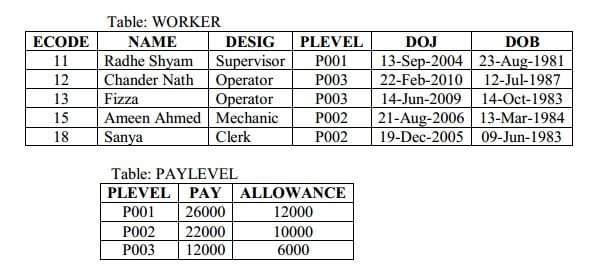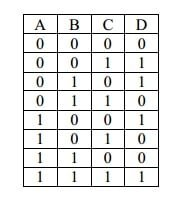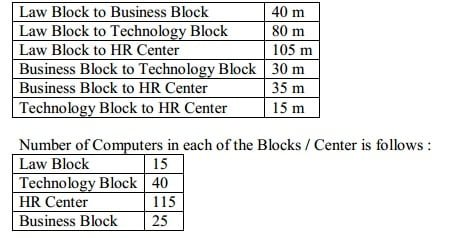Home » eVirtualGuru » Sample Paper of Computer Science (087) Class -12.

# Sample Paper of Computer Science (087) Class -12.

COMPUTER SCIENCE-2014

Time allowed: 3hours                                                                                                                                                  Maximum Marks: 70

Instructions (i) All questions are compulsory.

1.

(a)Define Multilevel and Multiple Inheritance with example .

(b) Write the names of the header files, which is/are essentially required to

run/execute the following C++ code:

void main ()

{

char C, String [] = “Excellence Overload”;

for (int I=0; String [I] ! = “\0‟;I++}

if (String [I] == “ “)

cout<<endl;

else

{

C=toupper(String [I]);

cout<<C;

}

}

(c) Rewrite the following program after removing the syntactical errors (if any).

Underline each correction.

#include [iostream.h]

typedef char Text (80);

void main ()

{

Text T=“Indian”;

int Count=strlen(T);

cout<<T<<„has‟<<Count<<„characters‟<<endl;

}

(d) Find the output of the following program:

# include <iostream.h>

void ChangeArray (int Number, int ARR[], int Size)

{

for (int L=0; L<Size; L++)

If (L<Number)

ARR[L] + = L;

else

ARR[L] * = L;

}

void Show(int ARR [], int Size)

{

for (int L=0; L<size; L++)

(L%2!=0) ? cout<<ARR[L] <<“#” : cout<<ARR[L] <<endl;

}

void main ( )

{

int Array [] = {30,20,40,10,60,50};

ChangeArray (3, Array, 6);

Show (Arry,6);

}

(e) Find the output of the following program:

# include <iostream.h>

void main ( )

{

int Track [] = {10,20,30,40,}, *Striker ;

Striker=Track ;

Track  + = 30 ;

cout<<“Striker>”<<*Striker<<endl ;

* Striker – =10 ;

Striker++;

cout<<“Next @”<<*Striker <<endl ;

Striker+=2 ;

cout<<“last @”<<*Striker<<endl ;

cout<<“Reset To” <<Track  <<endl ;

}

(f) Go through the C++ code shown below, and find out the possible output or output from the suggested output options (i) to(iv). Also, write the least value and highest value, which can be assigned to the variable guess.

# include <iostream.h>

#include <stdlib.h>

void main ( )

{

randomize ();

int Guess, High=4 ;

Guess=random (High) +50;

for (int C=guess ; C<=55 ; C++)

cout<<C<<“#”;

}

(i) 50 # 51 # 52 # 53 # 54 # 55 #

(ii) 52 # 53 # 54 # 55 #

(iii) 53 # 54 #

(iv) 51 # 52 # 53 # 54 # 55

2.

(a) Differentiate between members, which are present within the private visibility mode with those which are present within the public visibility modes.

(b) Write the output of the following C++ code. Also, write the name of feature of Object Oriented Programming used in the following program jointly illustrated by

the functions [I] to [IV].

#include<iostream.h>

void Print ( )                                                                       // Function [I]

{

for (int K=1;K<=60; K++) cout<< “-”;

cout<<endl;

}

void Print (int N)                                                               //Function[II]

{

for (int K=1;K<=N; L++) cout<<“*”;

cout<<endl;

}

void Print(int A, int B)                                                     //Function[III]

{

for(int K=1;K<=B;K++) cout<<A*k;

cout<<endl;

}

void Print(char T, int N)                                                                 // Function[IV]

{

for (int K=1;k<=N;K++) cout<<T;

cout<<endl;

}

void main( )

{

int U=9,V=4,W=3;

char C =‟@‟;

Print(C,V);

Print(U,W);

}

(c) Define a class candidate in C++ with following Description:

Private Members

A data member RNo (Registration Number) of type long

A data member Name of type string

A data member Score of type float

A data member Remark of type string

A member function AssignRem( ) to assign Remarks as per the Score

obtained by a candidate. Score range and the respective Remarks are

shown as follows:

Score Remarks

>=50 Selected

less than 50 Not selected

Public members

A function ENTER( ) to allow user to enter values for RNo, Name, Score & call function AssignRem() to assign the remarks.

A function DISPLAY( ) to allow user to view the content of all the data members.

(d) Answer the question (i) to (iv) based on the following:

class Student

{

int Rno;

char Name;

float Marks;

protected:

void result( );

public:

Student ( );

void Register ( ); void Display( );

};

class Faculty

{

long FCode;

char FName ;

protected:

float Pay;

public:

Faculty ( );

void Enter( );

void Show( );

};

class Course: public Student, private Faculty

{

long CCode ; char CourseName ;

char StartDate , EndDate ;

public:

Course( );

void Commence ( );

void CDetail ( );

};

(i) Which type of inheritance is illustrated in the above C++ code?

(ii) Write the names of all the data members, which is /are accessible from member function Commence of class Course.

(iii) Write the names of member functions, which are accessible from objects of class Course.

(iv) Write the names of all the members, which are accessible from objects of class faculty.

3.

(a) An array P  is stored in the memory along the column with each of its Element occupying 4 bytes, find out the location of P , if P is stored at 9000.

4

(a) What do you understand by Union ,Cartesian Product operations in

relational algebra?

(b) What is Primary Key?

(c) Consider the following tables WORKER and PAYLEVEL and answer (b) and (c)

parts of this question:Write SQL commands for the following statements:

(i) To display the details of all WORKERs, descending order of DOB.

(ii) To display NAME and DESIG of those WORKERs who’s PLEVEL is either P001 or P002

(iii)To display the content of all the WORKERs table, whose DOB is in  between „19-JAN-1984‟ and 18-JAN-1987‟.

(iv) To add a new row with the following : 19 ,  “Kishore‟, “Operator‟, “P003‟, “19-Jun-2008‟, “11-Jul-1984‟

(c) Give the output of the following SQL queries:

(i) SELECT COUNT (PLEVEL), PLEVEL FROM WORKER GROUP BY PLEVEL;

(ii) SELECT MAX(DOB), MIN(DOJ) FROM WORKER;

(iii) SELECT Name, Pay FROM WORKER W, PAYLEVEL P WHERE W.PLEVEL = S.PLEVEL AND P.ECODE<13;

(iv) SELECT PLEVEL, PAY+ALLOWANCE FROM PAYLEVEL WHERE PLEVEL = „P003‟;

5.

(a) Prove DeMorgan’s First Theorem. 4

(b) Write the equivalent Boolean Expression for the following Logic Circuit.(c) Write the POS form of a Boolean function F, which is represented in a truth

table as follows:(d) Reduce the following Boolean Expression using K-Map :

F(P, Q, R, S) = ∑ (0, 1, 2, 4, 5, 6, 8, 12)

6.

(a) What is Cyber Law ?

(b) Differentiate between BUS and STAR topology of networks.

(c) What is VoIP?

(d) Out of the following, identify client side script(s) and server side script(s).

(a) ASP                                                                 (b) Javascript

(c) VBscript                                                          (b) JSP

(e) Quick Learn University is setting up its Academic blocks at Prayag Nagar and   planning to set up a network. The university             has 3 academic blocks and one Human Resource Center as shown in the diagram below:Center to center distances between various block/center is as follows :(1) Suggest the most suitable place (i.e. Block / Center) to install the server of this university with a suitable reason.

(2) Suggest an ideal layout for connecting these blocks/center for a wired connectivity.

(3) Which device you will suggest to be placed/ installed in each of these blocks/center to efficiently connect all the computers with in these blocks / center. (4) The university is planning to connect its admission office in the closest big city, which type of network out of LAN, MAN or WAN will be formed? Justify your answer.

(f) Which of the following will come under Cyber Crime?

1. Theft of a brand new sealed pack laptop

iii. Modification in a company data with unauthorized access

1. Photocopying a printed report

7.

Name the header files for the following functions
(1) getc
(2) floor
(3) isalpha
(4) gets

(5) setprecision

(6)randomize()

(7)isalpha()

(8)getxy()

(9) Difference between Increment and Decrement Operator.The main objective of this website is to provide quality study material to all students (from 1st to 12th class of any board) irrespective of their background as our motto is “Education for Everyone”. It is also a very good platform for teachers who want to share their valuable knowledge.